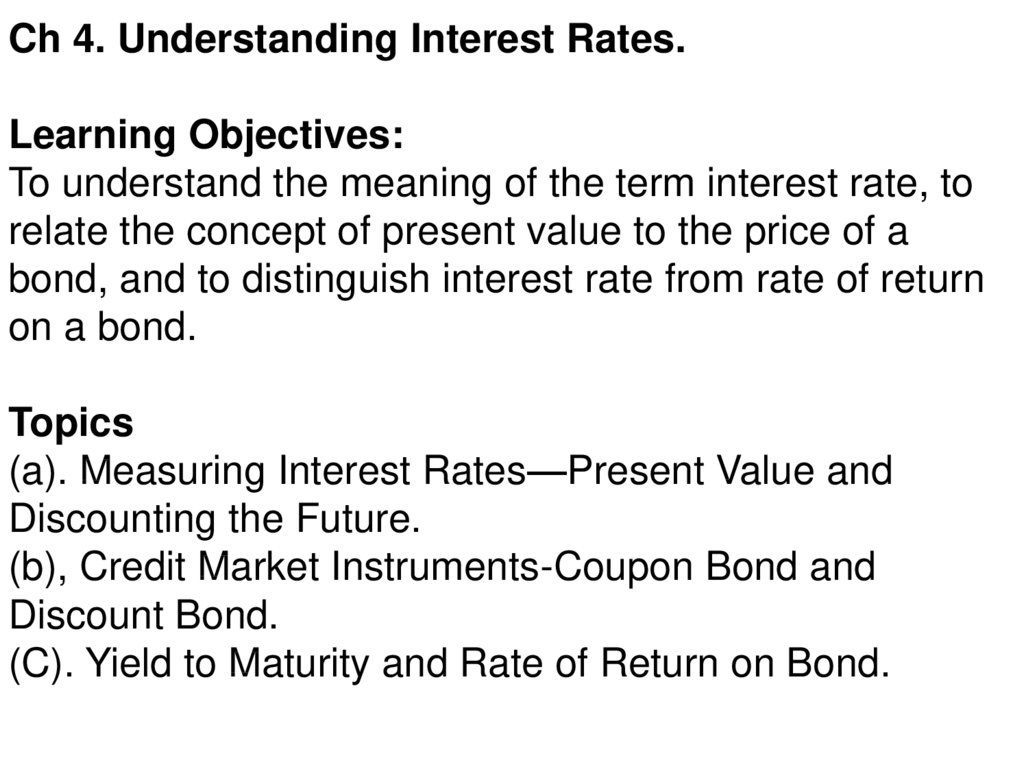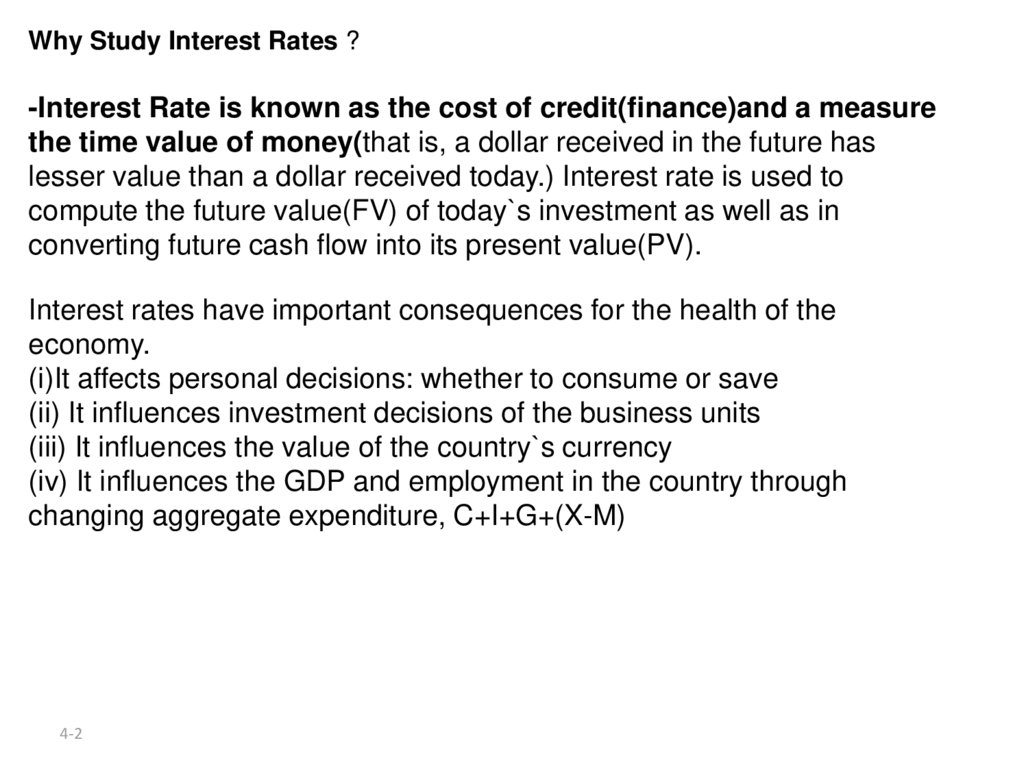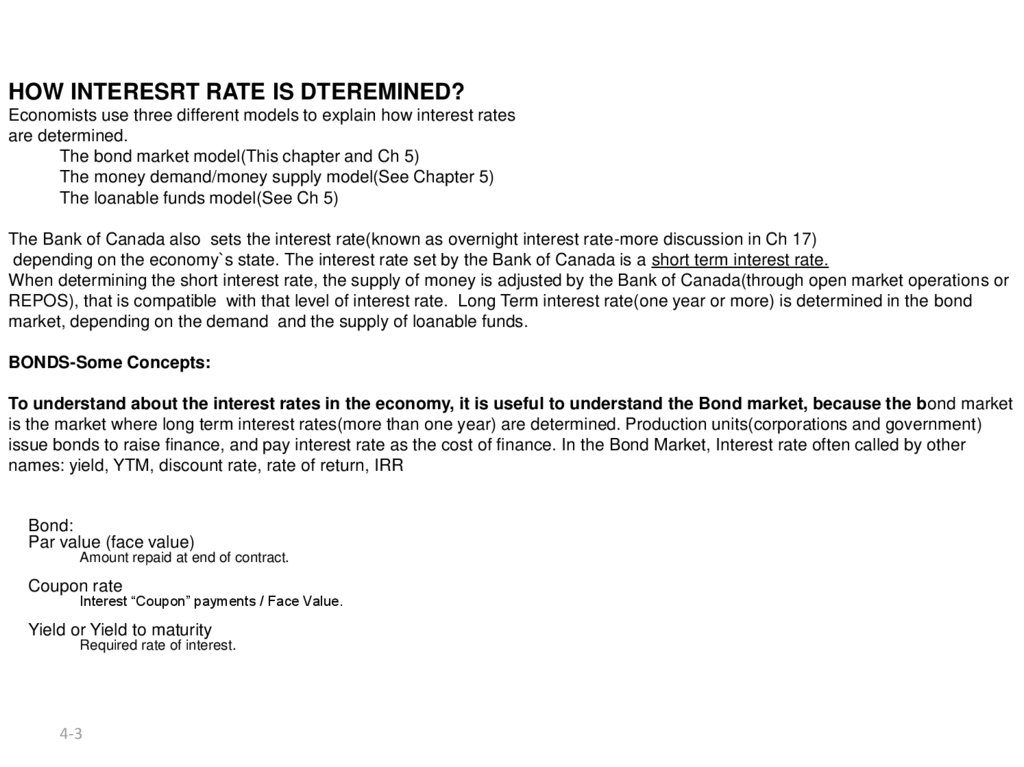# Understanding Interest Rates. Ch 4 Money Banking Revised

## 1.

Ch 4. Understanding Interest Rates.
Learning Objectives:
To understand the meaning of the term interest rate, to
relate the concept of present value to the price of a
bond, and to distinguish interest rate from rate of return
on a bond.
Topics
(a). Measuring Interest Rates—Present Value and
Discounting the Future.
(b), Credit Market Instruments-Coupon Bond and
Discount Bond.
(C). Yield to Maturity and Rate of Return on Bond.

## 2.

Why Study Interest Rates ?
-Interest Rate is known as the cost of credit(finance)and a measure
the time value of money(that is, a dollar received in the future has
lesser value than a dollar received today.) Interest rate is used to
compute the future value(FV) of today`s investment as well as in
converting future cash flow into its present value(PV).
Interest rates have important consequences for the health of the
economy.
(i)It affects personal decisions: whether to consume or save
(ii) It influences investment decisions of the business units
(iii) It influences the value of the country`s currency
(iv) It influences the GDP and employment in the country through
changing aggregate expenditure, C+I+G+(X-M)
4-2

## 3.

HOW INTERESRT RATE IS DTEREMINED?
Economists use three different models to explain how interest rates
are determined.
The bond market model(This chapter and Ch 5)
The money demand/money supply model(See Chapter 5)
The loanable funds model(See Ch 5)
The Bank of Canada also sets the interest rate(known as overnight interest rate-more discussion in Ch 17)
depending on the economy`s state. The interest rate set by the Bank of Canada is a short term interest rate.
When determining the short interest rate, the supply of money is adjusted by the Bank of Canada(through open market operations or
REPOS), that is compatible with that level of interest rate. Long Term interest rate(one year or more) is determined in the bond
market, depending on the demand and the supply of loanable funds.
BONDS-Some Concepts:
To understand about the interest rates in the economy, it is useful to understand the Bond market, because the bond market
is the market where long term interest rates(more than one year) are determined. Production units(corporations and government)
issue bonds to raise finance, and pay interest rate as the cost of finance. In the Bond Market, Interest rate often called by other
names: yield, YTM, discount rate, rate of return, IRR
Bond:
Par value (face value)
Amount repaid at end of contract.
Coupon rate
Interest “Coupon” payments / Face Value.
Yield or Yield to maturity
Required rate of interest.
4-3

## 4.

Interest Rate As A Time Value of Money.
Money has a time value because it can be invested to
make more money. Thus, a dollar received in the future
has lesser value than a dollar received today.
Conversely, a dollar received today is more valuable
than a dollar received in the future because it can be
invested to make more money. Formulas for the present
value and future value of money quantify this time value,
so that different investments can be compared.
Interest Rate is known as the cost of
credit(finance)and a measure the time value of
money(as represented by the PV or FV of
investments).

## 5. Time Value of Money :Time Line

We Cannot directly compare payments scheduled in different points in
the time line
Year
PV
\$100
\$100
\$100
\$100
0
1
2
n
100
100/(1+i)
100/(1+i)2
100/(1+i)n

## 6. Present Value(Time Value of Money)

• A dollar paid to you one year from now is less
valuable than a dollar paid to you today
• Why?
– A dollar deposited today can earn interest and
become \$1 x (1+i) one year from today.
– Due to the ongoing inflation, 1\$ today is not
equal to 1\$ after 1 year or in future
- Present wants and needs are more urgent than
future wants and needs. Human beings needs
compensation for impatience(or for this
Myopic-shortsightedness), otherwise people
will not save.

## 7.

Future Value (It is the idea of compounding)
FV of your \$100 lending for 2 years can be written as
FV=\$100×(1+0.10)2
In general term for n years, we can say
FV=PV(1+i)n
Present Value (It is the idea of discounting)
Discounting is the process of determining the present
value of a payment from a known future payment, or
future value.
PV = FV/(1+i)n...............

## 8. Applying the Present Value Concept to Credit Products We can apply the concept of Present value to four types of Credit Market

Instruments: Simple Loan, Fixed
payment loan, Coupon Bond and Discount bond. We
will focus here only on the Bond Market (the Coupon
and Discount bonds). In Bond market, the concept of
yield to maturity is the most accurate measure of
interest rates. In this chapter, we try to understand how
yield to maturity(on bond) is measured.
Audio slides on Calculating PRESENT VALUE
http://highered.mcgraw-hill.com/sites/0072946733/student_view0/chapter6/narrated_powerpoint_presentation.html
Audio slide on Bond Pricing
http://highered.mcgraw-hill.com/sites/0072946733/student_view0/chapter6/what_s_on_the_web_.html ]

## 9.

Example of Present Value and Yield to Maturity: A
Case of Coupon Bond
If you buy a bond maturing in n years, and you are assured that
the bond purchased at a price P would give you in each year a
coupon payment (C), and at the end of the maturity period of n
years when you return the bond you get the face value of the
bond plus coupon payment for the nth year as well, we have the
formula:
P = C/(1+i) + C/(1+i)2 + C/(1+i)3 +.....+ C/(1+i)n + F/(1+i)n
Where
P=Price of bond
C=Yearly Coupon payment
F=Face value
n=years to maturity date
i= discount rate(yield to maturity)

## 10. Yield to Maturity: Bonds

Coupon Bond (Coupon rate = 10% = Coupon payment/F, where F is a face value).
P=
\$100
+
(1+i)
\$100
+
(1+i)2
\$100
+ ... +
(1+i)3
\$100
+
(1+i)10
\$1000
(1+i)10
P=
C
+
(1+i)
C
+ 2
(1+i)
C
+ ...3 +
(1+i)
C
+ n
(1+i)
F
(1+i)n
Yield to maturity (i) in coupon bond case, represents the discount rate which equates the discounted value of a
bond's future cash flows(the right hand side after equal sign) to its current market price, P (left side in the above
equation).
For discount bond case, see below how i is calculated:
4. Discount Bond (P = \$900, F = \$1000), one year
\$900 =
\$1000
(1+i)
i=
\$1000 – \$900
\$900
i=
F–P
P
4-10
= 0.111 = 11.1%

## 11.

Taking a numerical example.
Consider a bond issued by the Government of Canada, which
pays 10% coupon with a face value of \$1000, and 8 years to
maturity. Assume that the discount rate,i, is 10%(which means
yield to maturity is 10%), and the market price of the bond is
\$1000, then we can write(using the PV formula)
\$1000 = \$100/(1+0.10) + \$100/(1+0.10)2 + \$100/(1+0.10)3 +.....+
\$100/(1+0.10)8 + \$1000/(1+0.10)8 ..........................(5)
Now suppose the discount rate(Yield to Maturity) changes from
10% to 12.25%, what would be the market price, P, of the above
bond? We find now that
\$ 889.1977 = \$100/(1+0.1225) + \$100/(1+0.1225)2 +
\$100/(1+0.1225)3 +.....+ \$100/(1+0.1225)8 + \$1000/(1+0.10)8
http://www.studyfinance.com/templates/bondvaluation.xls

## 12.

On the basis of the previous slides, we can draw the following
conclusions:
(*)
(a) Yield to maturity(YTM) is the discount rate(interest rate) that
equates the present value of payments(in the above case, coupon
payments for 8 years, and the face value at the end of 8th year)
from a the coupon bond with its market price(value) today.
(b) When the coupon bond is pried at its face value, the yield to
maturity equals the coupon rate(which is 10% in our example).
We say that in this case bond is selling at par.
(c) When the yield to maturity is greater than the coupon rate, the
bond price (market value) is below its face value. In this case,
bond is selling at discount.
(d) It can be shown that when the yield to maturity is less than the coupon rate,
the bond price (market value) would be above its face value. In this case, bond

## 13. Relationship Between Price and Yield to Maturity

Three Interesting Facts in Table 1
1.
When bond is at par, yield equals coupon rate(or when coupon bond is
priced at its face value, the yield to maturity equals coupon rate);
2.
Price and yield are negatively related;
3.
Yield to maturity is greater than coupon rate when bond price is below
par value;
4-13

## 14. Bonds Premiums & Discounts

Bonds
What happens to bond values if required return is not equal to the coupon rate?
The bond's value will differ from its par value.
4-14
R > Coupon Interest Rate
P0 < par value
=
DISCOUNT
R < Coupon Interest Rate
P0 > par value
=

4-15

4-16

## 17.

Bond dealers BUY at the BID price and SELL at the ASKED price, the difference is the
commission. They buy low and sell high.
As customers, we would buy at the ASKED price and sell to the dealer at the BID price.
Bid Price (Wholesale price)-Price you receive when you sell bond.
Yield column is the YTM
YTM ≈Current yield, when bonds maturity is more than 20 years.
In our example, Current yield for the Canada bond 4, maturing in 2029,
is within one basis point of the value for maturity. (5.69% - 5.68%)
Tbills -4-17
Quoted as yields, not prices

4-18

## 19. Other Measures of Interest rate: (a)Current Yield

ic =
C
P
Two Characteristics
1. Ic better approximation to yield to maturity, nearer price is to par and longer is
maturity of bond
2. Change in current yield always signals change in same direction as yield to maturity
(b)Yield on a Discount Basis
idb =
(F – P)
x
P
365
(number of days to maturity)
A 91-day bill, P = \$988, F = \$1000
idb =
\$1000 – \$988
\$988
x
365
91
= 0.0487 = 4.8%
Two Characteristics
1. Understates yield to maturity
2. Change in discount yield always signals change in same direction as yield to maturity
4-19

## 20.

(C)Coupon rate
For a bond that pays interest payments on a periodic basis is
known as a coupon bond. Each coupon bond specifies a coupon
rate that is expressed as a percentage of the face value of the
bond.
For example, a coupon bond with a 10% coupon rate will pay the
holder a \$100 a year if the face value is \$1,000. The coupon rate
is predetermined and it is not affected by any economic conditions
once the bond is issued.

## 21.

Rate of Return on a Coupon Bond
There is a distinction between yield to maturity, rate of return and
coupon rate.
The Return on Bond, when it s held from time t to t+1, is
composed of two elements: (i) interest(coupon) payments
received during this period, and (ii) the capital gains earned by
disposing the bond.
The return on a bond held from time t to t+1 can be expressed as
RET= {C + (Pt+1 - Pt)}/Pt
where RET= return in % from holding the bond from time t to t+1
C
= coupon payment
Pt
= price of the bond paid at time t
Pt+1 = price of the bond at time (t+1)

Rate of Return:
RET =
C + Pt+1 – Pt
Pt
where: ic =
g=
4-22
C
Pt
Pt+1 – Pt
Pt
= ic + g
= current yield
= capital gain

4-23

## 24. Maturity and the Volatility of Bond Returns.

Key Findings from Table 2
1. Only bond whose return = yield is one with maturity = holding period.
2. For bonds with maturity > holding period, i P implying capital loss.
3. Longer is maturity, greater is % price change associated with interest rate
change.
4. Longer is maturity, more return changes with change in interest rate.
5. Bond with high initial interest rate can still have negative return if i .
Conclusion from Table 2 Analysis
1. Prices and returns more volatile for long-term bonds because have higher
interest-rate risk.
2. No interest-rate risk for any bond whose maturity equals holding period.
4-24

## 25. Distinction Between Real and Nominal Interest Rates.

Real Interest Rate:
Interest rate that is adjusted for expected changes in the price level
ir = i – e
1. Real interest rate more accurately reflects true cost of borrowing.
2. When real rate is low, greater incentives to borrow and less to lend.
if i = 5% and e = 3% then:
ir = 5% – 3% = 2%;
if i = 8% and e = 10% then
ir = 8% – 10% = –2%;
4-25

## 26.

NOTE: This is not an examinable content. This is Optional to learn.
Source: http://www.studyfinance.com/templates/bondvaluation.xls
Market Price of Bonds can be easily calculated using the Present Value function in Excel.
This function is labelled PV
and listed under the financial category of functions in excel. The inputs are similar to a financial calculator. The following is an
example of calculation the price(PV) of a \$1000 bond(face or value at maturity), maturing after 10 years(with 20 semi-annual
payments) and carrying an annual coup[on of 12%(6% semi-annual)
Rate is the yield of the bond per period. In the case of this bond it
has a annual yield of 12% and a semiannual yield of 6%.
Nper is the total number of periods. This is a 10 year bond
compounded semiannually, therefore there are 20 periods.
Pmt is the coupon payment per period. This bond has an annual
coupon payment of 10% or \$100 and a semiannual coupon of 5%
or \$50.
Fv is the future value or face value of the bond. This bond has a
face value of \$1000.
Type is used to define the timing of the payments. If the
payments are made at the beginning of the period enter 1. If the
payments come at the end of the period leave blank or enter 0.
Coupon payments typically come at the end of the period.
Note that the present value is negative while the payment and
future values are positive. The present value is negative because
this is a payment for the bond, and the future value and payments
are positive because these are payments you receive.
4-26

## 27.

NOTE: This is not an examinable content. This is Optional to learn.
Bond Yield(YTM) Using Excel
Bond yields can be easily calculated using the rate function in Excel. The Rate function can be found under the financial
category. The inputs are similar to a financial calculator.
Bond Yield(YTM) Example:
Calculate the yield to maturity on a 6 year bond with a 5% coupon, a market value of \$990, and a face value of \$1000.
Nper is the total number of periods. This bond matures in 6
years.
Pmt is the annual coupon payment. This bond pays 5%
annually and it has a face value of \$1000. The annual coupon
payment is \$50.
Pv is the present value or market value of the bond. This bond
has a market value of \$990.
Fv is the future value or face value of the bond. This bond has a
face value of \$1000
Type is used to define the timing of the payments. If the
payments are made at the beginning of the period enter 1. If the
payments come at the end of the period leave blank or enter 0.
Coupon payments typically come at the end of the period.
Note that the present value is negative while the payment and
future values are positive. The present value is negative
because this is a payment for the bond, and the future value and
payments are positive because these are payments you receive.
4-27